# Two Zener diodes (A and B) having breakdown voltages

Question:

Two Zener diodes (A and B) having breakdown voltages of $6 \mathrm{~V}$ and $4 \mathrm{~V}$ respectively, are connected as shown in athe circuit below. The output voltage $\mathrm{V}_{0}$ variation with input voltage linearly increasing with time, is given by:

$\left(\mathrm{V}_{\text {input }}=0 \mathrm{~V}\right.$ at $\left.\mathrm{t}=0\right)$ (figures are qualitative)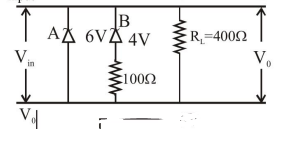1.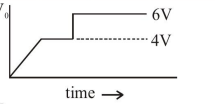2.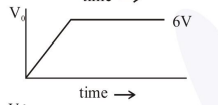3.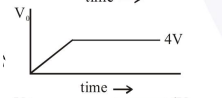4.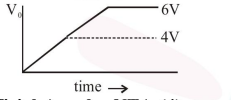Correct Option: , 4

Solution:

Till input voltage Reaches $4 \mathrm{~V}$ No zener is in Breakdown Region So $\mathrm{V}_{0}=\mathrm{V}_{\mathrm{i}}$ Then Now when $\mathrm{V}_{\mathrm{i}}$ changes between $4 \mathrm{~V}$ to $6 \mathrm{~V}$ One Zener with 4V will Breakdown are P.D. across This zener will become constant and Remaining Potential will drop. acro

Resistance in series with 4V Zener.

Now current in circuit increases Abruptly and source must have an internal resistance due to which. Some potential will get drop across the source also so correct graph between $\mathrm{V}_{0}$ and t. will be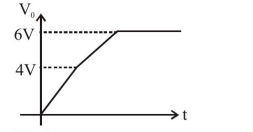We have to Assume some resistance in series with source.